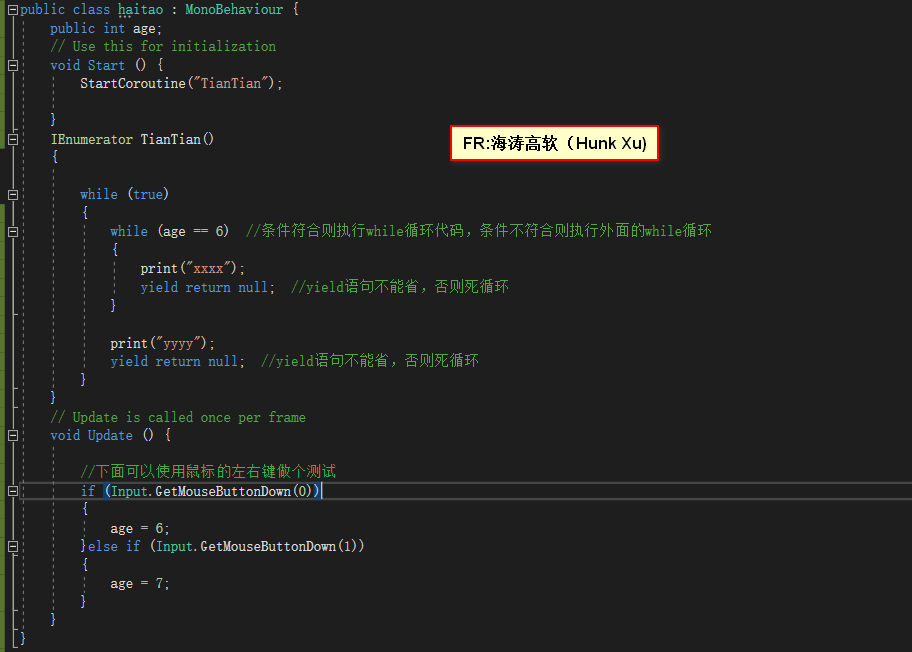• SQL没有for循环，没有数组概念。 SQL可以用关键字order by排序。 declare @str varchar(500), @Count int, @i int, @j int, @num1 int, @num2 int, @temp int --接收一串字符 SET @str='98 108 102 91...
SQL没有for循环，没有数组概念。

SQL可以用关键字order by排序。

declare

@str varchar(500),

@Count int,

@i int,

@j int,

@num1 int,

@num2 int,

@temp int

--接收一串字符

SET @str='98 108 102 91 89 88 98 91 97 102'

--按空格拆开插入表中,把' '替换成' union all select '

SELECT @str=REPLACE(@str,' ',' union all select ')

--创建临时表

CREATE TABLE table1 (ID INT IDENTITY(1,1),num int)

--处理SQL语句

SELECT @str='insert into table1 (num) select '+@str

--执行SQL语句

EXEC(@str)

IF exists(select * from table1)

begin

--一共有多少个数（用来计算需要循环多少次）

select @Count=Count(num) from table1

--开始循环

set @i=1

--外循环

--只知道一个数一共要比较几次（比如有4个数,第一个要比较3次,第二个要比较2次,第三个比较1次）

--知道了比较次数,然后才具体做排序（内循环）

while (@i<@Count)

begin

--内循环while结束后，开始第二次外循环，重新开始，重新赋初值（像for(int @j=@i...)）

set @j=@i

--内循环

--具体比较的过程（比如要比较3次,第一次和第2个数比较,第二次和第3个数比较,第三次和第4个数比较）

while (@j<@Count)

begin

--要比较的第一个数（第一个数不变）

select @num1=num from table1 where ID=@i

--要比较的第二个数（第二个数按次数增加）

select @num2=num from table1 where ID=@j+1

if @num1>@num2

begin

--交换数字

--把大的数先放一边

set @temp=@num1

--把小的数放到前边

update table1

set num=@num2

where ID=@i

--把大的放后边

update table1

set num=@temp

where ID=@j+1

end

set @j=@j+1

end

set @i=@i+1

end

end

select * from table1

drop table table1

GO

-----------------------------------------------------------展开全文• 执行结果：鼠标左键点击，不停的打印xxxx鼠标右键点击，不停的打印yyyy------------------------------------------------由此可见 在携程中嵌套两个while循环可以做状态的切换------------------------------------...执行结果：鼠标左键点击，不停的打印xxxx鼠标右键点击，不停的打印yyyy------------------------------------------------由此可见 在携程中嵌套两个while循环可以做状态的切换------------------------------------------------FR:海涛高软（QQ技术交流群：386476712）
展开全文• while(true){ //输出当前处理结果 //将index定位在起点 while(未将所有index处理完毕) { if(当前index没处理完) { //处理当前index break; } else {
while(true)
{
//输出当前处理结果
//将index定位在起点
while(未将所有index处理完毕)
{
if(当前index没处理完)
{
//处理当前index
break;
}
else
{
//当前index已经处理完毕
//重新初始化当前index 移动当前index
}
}
if(所有index都已经处理完毕)
break;
}
这个两层的while循环可以代替n层for循环使用，n就是index的取值范围宽度。从而在问题规模即n发生改变时，不必多加一层for，而只修改n即可。
内层while循环负责的if分支负责对当前index进行遍历，每次执行if分支都会break，然后就又回到外层while循环中进行处理。
当当前index遍历完毕，便走向else分支，此时移动index，相当于n层for循环结构中的某内层for循环遍历完毕，程序暂时回到其外层for循环。else分支并不break，留在内层while里，于是再次来到if分支，对当前已经移动的index进行遍历，由于对于此index（相当于外层index）进行遍历的时候，每一次移动还要包含对内层index的遍历，于是在break回到外层while后，会将index重新定位在起点，即最内层。
如此往复。
展开全文• #python中，while语句用于循环执行程序，即在某个条件下，循环执行某段程序，以处理需要重复处理的相同任务。#while是“当型”循环结构。i=1while i<=20:print(i,end=" ")i+=1sum=0i=1while i<=100:sum+=ii+=1...
#python中，while语句用于循环执行程序，即在某个条件下，循环执行某段程序，以处理需要重复处理的相同任务。#while是“当型”循环结构。i=1while i<=20:print(i,end=" ")i+=1sum=0i=1while i<=100:sum+=ii+=1else:print("\n",sum)print("0+2+...+100=",sum)#从控制台录入一个数字，求从1到数字的阶乘；result=1;i=1;numA=0;numA=int(input("请输入一个数字："))while i<=numA:result*=ii+=1else:print("循环结束!")print("结果是：",result)#使用while循环输出100以内的素数x=2while x<100:n=2while nif x%n==0:breakn+=1else:print(x,end="  ")x+=1else:print("循环结束")#输出100之内能被7整除的数nNum=7;lineCount=0;while nNum<=100:if nNum%7 !=0:nNum+=1;continueelse:print(nNum,end="  ")lineCount+=1if lineCount==5:print()  #单个print()换行;lineCount=0nNum+=1;else:print("\n循环结束")#使用while循环计算从1到100之和sum=0;i=1;while i<=100:sum+=i;i+=1;else:print("1+2+3+...+100=",sum);print("\n程序结束")#从控制台录入打印符号的数量。row=0;i=0;row=int(input("请输入行数："));while iprint()print("",end="  ")i+=1;else:print("\n程序运行结束")#使用while循环和for循环嵌套.python语言允许在一个循环体中嵌套另外一个循环体。#实例：从控制台输入行数和列数，打印正方形。i=1;j=1;numA=int(input("请输入行数："))numB=int(input("请输入列数："))while i<=numA:j=1while j<=numB:print("*",end="  ")j+=1print()i+=1#使用while循环嵌套打印九九乘法口诀表;i=1;j=1;while i<=9:j=1while j<=i:print("%d%d=%2d"%(j,i,ij),end="       ")j+=1print()i+=1else:print("\n程序运行结束")#使用for循环打印九九乘法口诀表;for i in range(1,10):for j in range(1,10):if j<=i:print("%d%d=%2d"%(j,i,ij),end="     ")else:print()else:print("\n程序运行结束");
展开全文• 循环结构  ：当重复执行相同的代码或者是相似的代码时。 循环三要素：  1): 循环变量的声明:用于控制循环次数的循环因子  2): 循环条件: 用于判断是否执行相同或相似内容(循环体)的条件  3)：循环变量的改变...
• #python中，while语句用于循环执行程序，即在某个条件下，循环执行某段程序，以处理需要重复处理的相同任务。#while是“当型”循环结构。i=1while i<=20:print(i,end=" ")i+=1 sum=0i=1while i<=100:sum+=ii+=...
• 描述：双层while循环时，想在不满足外层循环条件时跳出内层循环 上面代码中的第二个方法中，如果内层循环中两个while循环不添加条件left<right。使用debug的时候，当left指向t，right指向c时，此时，满足外层...leetcode js 指针 javascript
• 双层while循环：（使用时应注意判断条件和自增（自减）变量的位置） # 打印九九乘法表 i = 1 while i <= 9 : j = 1 while j <= i: print( "%d * %d = %2d " % (i, j, i * j) , end = "") j += 1 print...
• 当使用双层while循环时，想在不满足外层循环条件时跳出内层循环时需要在内层循环条件添加外层循环条件，如下代码所示，因为当满足while条件时进入while循环体内，在没有中止或退出循环语句的情况下需要至少执行一次...python
• 本文主要介绍海克斯康三坐标PC-DMIS软件使用赋值、循环、判断语句...以下是PC-DMIS使用双层DO...UNTIL循环语句实现测量的过程！文章主题包括：视频分享测量程序分享与讲解测量程序下载作者在这里抛砖引玉，供读者玩...
• 1.for循环'''循环可能会被用来破坏攻击别人的网站for 变量名 in 集合:语句'''#range(i, j) produces i, i+1, i+2, ..., j-1.print(range(8))# print('hello\n'*20)for i in range(8):print(i)'''单词：Convert 转换...
• 目标：使用双层嵌套循环完成小星星的输出 三种方法，输出一行的小星星 # 以三个星星为例 print('*'*3) # 三次print一个星 ...# 用while循环来完成 count = 0 # 计数器，初始条件 while count < 3: pri...
• #### 双层循环练习#十行十列小星星j =0while j<10:#逻辑代码写在下面#打印一行十个小星星i =0while i<10:print("*",end="")i+=1#打印换行print()j+=1#十行十列隔列换色小星星j =0while j<10:#打印星星i=0...
• 原标题：Python基础 | 关于“循环”那些事for 循环listrangeenumeratezipwhile 循环while相当于if时while充当for和if的混合体泛循环列表解析map迭代器生成器循环的跳出和继续单层循环嵌套循环Python中常见的循环有两...
• Python中九九乘法表从四个不同方向打印的方法：这里用while循环来实现，双层循环是必须的 #左下角九九乘法表： i=1 while i < 10: j=1 while j <= i: print("%d*%d=%2d "%(j,i,i*j),end="") #控制输出...
• # 其他语言用break2 就能退出两层循环 # 用标记变量退出 flag=True while flag: for x in range(10): print(x) flag=False break # 使用函数配合return 语句实现 def test(): while True:
• Java双层for循环 数学黑洞6174 Scanner input=new Scanner(System.in); System.out.print("请输入一个任意的四位正整数："); int a=input.nextInt(); int min,max;//最大值 最小值 int b=0;//记次数 int[] ...java
• 1.while.....else的使用 在 while … else 在条件语句为 false 时执行 else 的语句块。 语法格式如下： while <expr>: <statement(s)> else: <additional_statement(s)> 功能： ...
• python学习 day6双层循环练习十行十列小星星十行十列隔列换色小星星十行十列隔行换色小星星99乘法表方向一方向二方向三方向四求吉利数字方法一方法二 字符串形式百钱买百鸡 双层循环练习 本次课题：利用双层循环...python
• 接下来看双层循环循环嵌套是指在一个循环语句中再定义一个循环语句的语法结构。     while、do.. while和for循环语句都可以进行嵌套，并且他们之间可以进行互相嵌套。最常见的是在for循环中嵌套for循环。...
• for循环单层循环执行流程：http://blog.csdn.net/asheandwine/article/details/76383304接下来看双层循环循环嵌套是指在一个循环语句中再定义一个循环语句的语法结构。while、do.. while和for循环语句都可以进行...
• 本文将讲述单层循环双层循环，以及日期循环单层循环测试表代码create table test(location_id int ,location_name varchar(10));再编写存储过程，其中涉及到循环的使用。我们欲通过这个存储过程，来达到往表中...
• # (1) 99 乘法表 方向一i = 1while i<=9:# 打印表达式j = 1while j <= i:print("%d*%d=%2d " % (i,j,i*j) ,end="")j+=1# 打印换行print()i+=1# 方向二i = 1while i<=9:# 打印空格k = 9 - iwhile k>0:...
• 1.删除链表中的重复元素，要求是不能够使用缓冲区 由于题目要求了不能够使用额外的空间来进行辅助...在链表中一般是使用while循环而不使用for循环来遍历元素 其中要使用到四个指针，第一个指针first指向的是第一...
• 双层循环练习 #里面一层是一行十列 外层加上十行 1.1 十行十列小星星 j=0 while j<10: i = 0 while i < 10: print(‘★’, end=’’) i += 1 print() j+=1 1.2 十行十列隔列换色小星星 j=0 while j<10: i...
• 展开全部回答即可62616964757a686964616fe59b9ee...Turbo Pascal采用不同的循环方式来实现，常用的环循有三种: for、repeat、while. 第一节 for 循环for循环是一种自动计数型循环。[例3.1] 试打印出1~20的自然数。解...
• while i<=9: # 打印表达式 j = 1 while j <= i: print("%d*%d=%2d " % (i,j,i*j) ,end="") j+=1 # 打印换行 print() i+=1 # 方向二 i = 1 while i<=9: # ...
• 批量插入Mysql数据 delimiter $$DROP PROCEDURE IF EXISTS pre1; ## 判断当前存储过程是否存在...WHILE i < 1000 DO INSERT INTO class(id, name) VALUES (i, '班级'); SET i = i+1; END WHILE; END$$ CALL pr
• 文章目录1、99乘法表矩形正直角三角形反直角三角形倒直角三角形等...while 语句： #!/bin/bash # 99乘法表 a=1 while [ $a -le 9 ] do b=1 while [$b -le $a ] do c=$[$a*$b] echo -ne "$a*$b=\$c\t" let b++shell linux